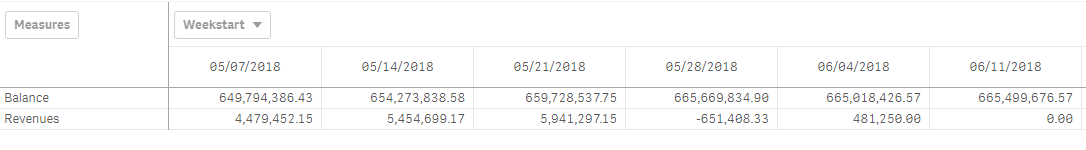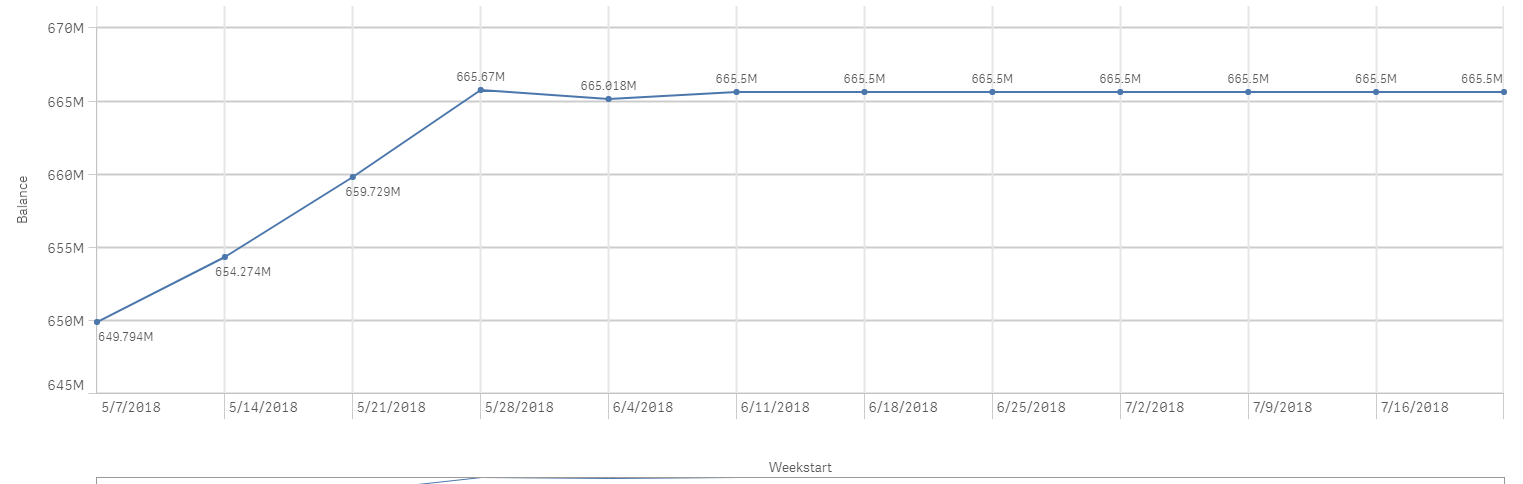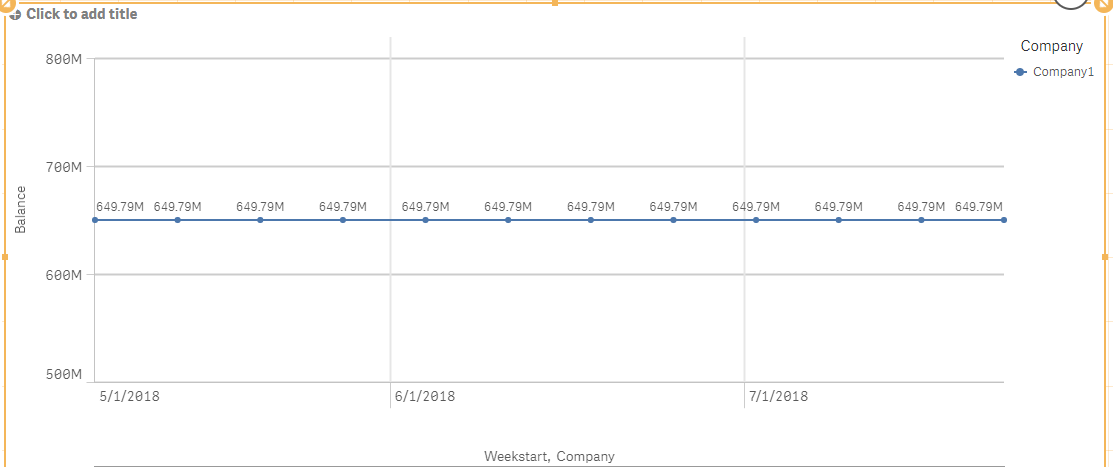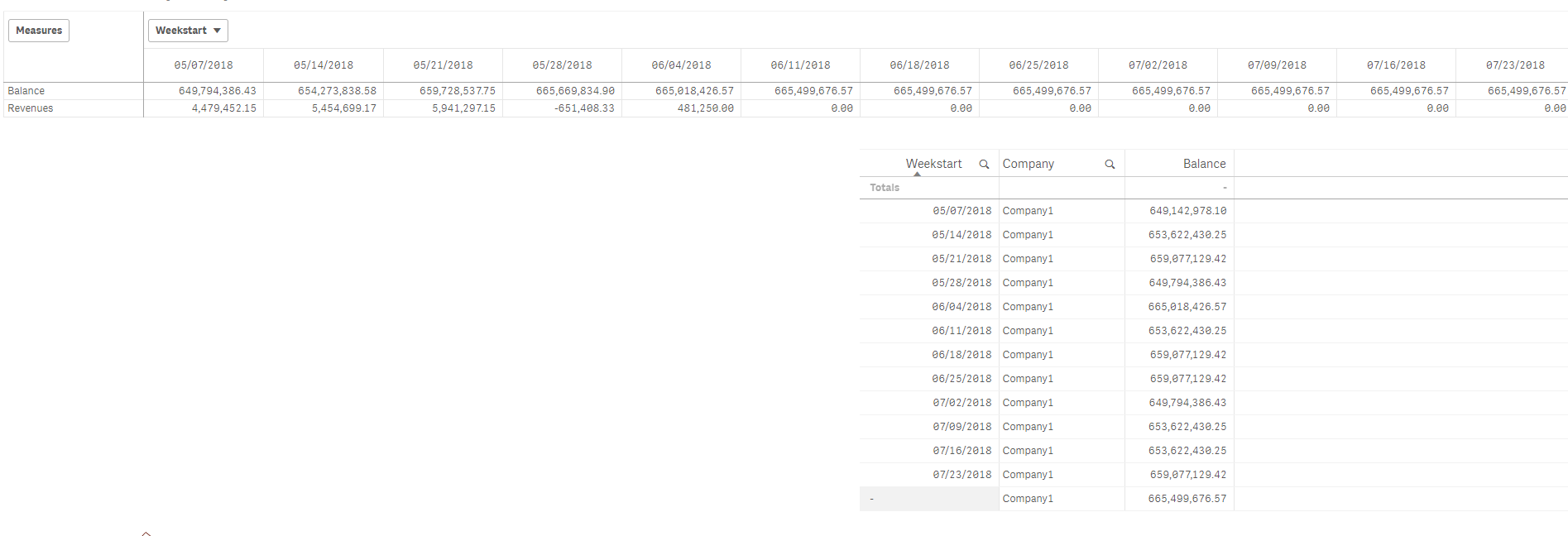Announcements
Applications are open for the 2024 Qlik Luminary Program. Apply by December 15 here.
cancel
Showing results for
Did you mean:Creator III

## Rangesum Above with Multiple Dimensions

Hi All,

I have the below pivot tableThe balance is the previous weeks balance plus the previous weeks revenues which I get using the expression

RangeSum(

Sum(TOTAL {<[Weekstart]>} Balance),

RangeSum(Before(Sum({<[ Weekstart]>}UnsettledSell)

, 1, ColumnNo())))

I then have appended this expression to the following for use in a line chart and it works great.

RangeSum(

Sum(TOTAL {<[Weekstart]>} Balance),

RangeSum(Above(Sum({<[ Weekstart]>}UnsettledSell)

, 1, RowNo())))The problem I am having is I want to add another dimension 'Company' to the line chart it ruins the expression and gives me the below.I believe it has to do with the above function and multiple dimensions but I am unsure on how to solve.

Thanks!!

Mark

Labels (1)
• ### function

1 Solution

Accepted SolutionsMVP

Are you sure you used this?

Aggr(

RangeSum(

Sum(TOTAL <Company> {<[Weekstart]>} Balance),

RangeSum(Above(Sum({<[ Weekstart]>}UnsettledSell)

, 1, RowNo())))

, Company, (Weekstart, (NUMERIC)))

6 RepliesMVP

May be try this

Aggr(

RangeSum(

Sum(TOTAL <Company> {<[Weekstart]>} Balance),

RangeSum(Above(Sum({<[ Weekstart]>}UnsettledSell)

, 1, RowNo())))

, Company, Weekstart)

or this

Aggr(

RangeSum(

Sum(TOTAL {<[Weekstart]>} Balance),

RangeSum(Above(Sum({<[ Weekstart]>}UnsettledSell)

, 1, RowNo())))

, Company, Weekstart)Creator III
Author

Not quite working, the balances seem to be applied to the incorrect weeks.

The pivot table shows the desired results and the straight table shows what I am gettingMVP

Aggr(

RangeSum(

Sum(TOTAL <Company> {<[Weekstart]>} Balance),

RangeSum(Above(Sum({<[ Weekstart]>}UnsettledSell)

, 1, RowNo())))

, Company, (Weekstart, (NUMERIC)))Creator III
Author

Closer I think! That works great when I have one company selected, however if I select a second company the starting balance for each company becomes the sum of their two starting balances. Their revenues are then applied appropriately in subsequent weeks but the starting balance is the sum of the two companies.MVP

Are you sure you used this?

Aggr(

RangeSum(

Sum(TOTAL <Company> {<[Weekstart]>} Balance),

RangeSum(Above(Sum({<[ Weekstart]>}UnsettledSell)

, 1, RowNo())))

, Company, (Weekstart, (NUMERIC)))Creator III
Author

Ah I was not, it works great thank you!!Tags
Community Browser